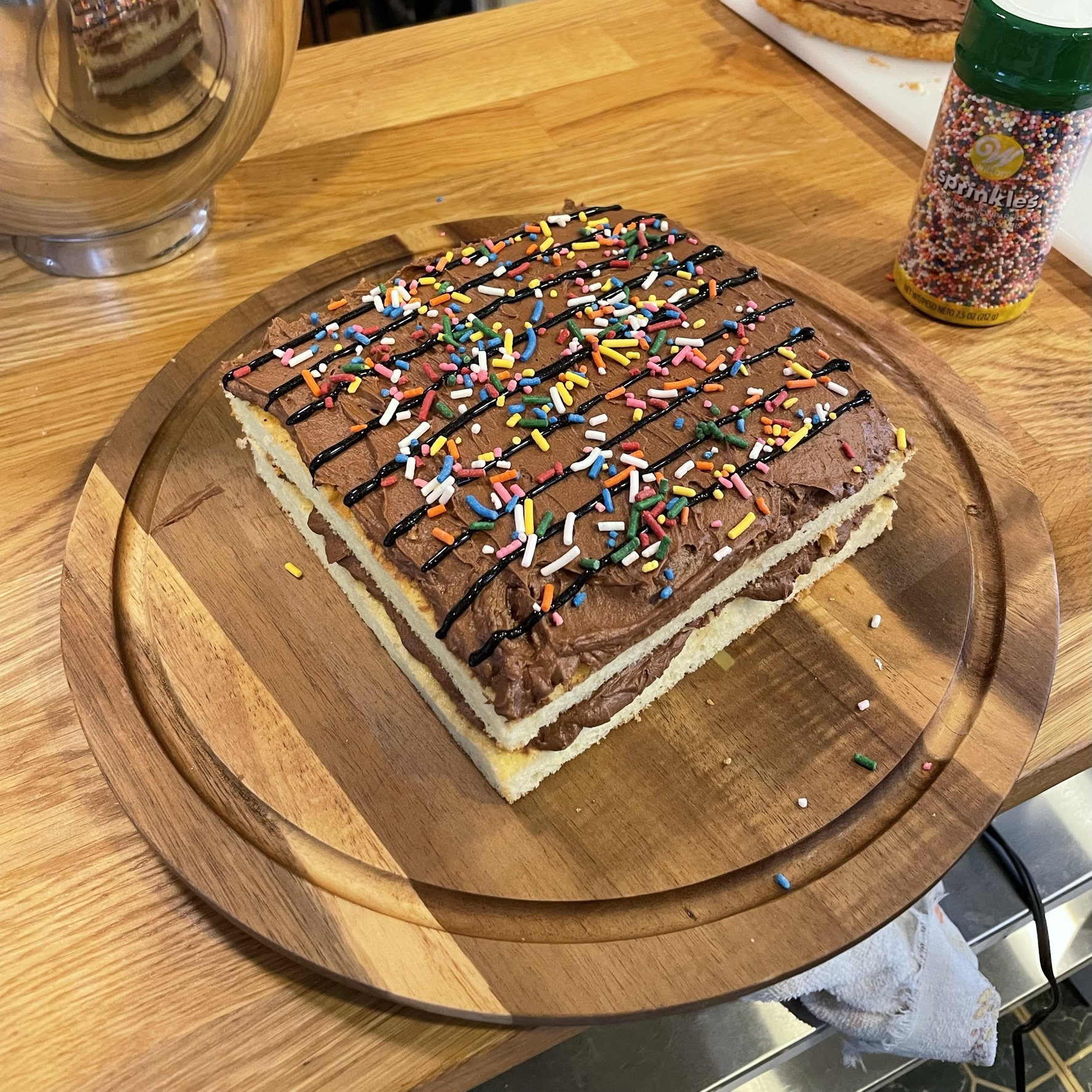# Approximating pi using... a cake?

Tuesday, March 14, 2023

Happy Pi Day, fellow nerds! This is a holiday I've celebrated every year since at least 2010, and I'm not stopping anytime soon.

The celebrations have evolved. It used to be just "bake a pie" and "haha pi, pie".

Over time, I twisted it a bit (pizza is a pie of sorts! a cake with a pi symbol on it!). This year is the next evolution. I've made a cake with an experiment on it for estimating the value of pi.

This is a really cool technique called Buffon's needle problem and I first heard about it from my grandfather at a restaurant. I think I was in middle school. Anyway, he was telling me about this way that you could estimate pi by tossing a needle on the floor and counting the number of times where it ended up crossing the line between floor boards.

I didn't really get it then, but it stuck in my mind that it was really neat that you could do this thing to estimate the value of pi! I understood it had something to do with the needle being able to form a circle (rotated around its center) and some such.

Fast forward to 2023, and I'm sitting idly thinking about Pi Day plans, and I realize. I can make a cake. I can draw lines on it. I have sprinkles. We can do Grandpa Bill's pi needle estimate, but on a cake!

First, I have to figure out what is that even that he had told me about. It was easy enough to find the Wikipedia page for Buffon's needle problem. The original formulation wasn't around estimating the value of pi, but it sure can be used that way.

Basically, you have this formula: `p = (2/pi) * (l/t)`, where:

• `p` is the probability that the needle will cross the line between two floor boards
• `l` is the length of the needle
• `t` is the width of the floor boards

We can reformulate this as `pi = (2/p) * (l/t)`, and then can derive an estimate of pi from an estimate of the probability that the needle crosses a floor board. Or the probability that a sprinkle crosses a line on a cake. You see where this is going.

We're going to "bake" a cake on an HTML canvas, and do a Monte Carlo simulation of the value of pi.

The first thing we need to do is setup our canvas. We make the element, and set some styles so that it's square and as big as can be, but not too big, we're not monsters.

``````<canvas id="needles" style="aspect-ratio: 1/1; display: inline-block; width: 100%; max-width: 400px;"></canvas>
``````

Then we do a little bit of JS to make the canvas scale to the size of the element. We add the lines on the cake, and we add sprinkles on it.

The code is all available in the repo, so I won't go into detail on all of it here. But there's this one really cool bit I ran across while coding it up.

How do you put the sprinkle facing a random direction? My first thought was to generate a random angle and then compute the sprinkle vector from there. That either relies on picking an angle in radians (thus relying on pi) or using sine or cosine, which also feels like it's against the spirit of estimating pi. So what to do?

Enter: the unit circle!

I found a cool blog post which mentioned an algorithm from von Neumann himself. The key insight is that if you have a uniformly distributed random number in a range, you can map that onto the unit circle (and keep regenerating if you are outside the unit circle). Then you can scale it to land on the circle, instead of inside it, and you now have a random point on the circumference of the unit circle!

Let's see that in code.

``````// Generate a vector at a random angle between -90 and 90 degrees
function randomAngleUnitVector() {
// If we're not inside the unit circle, we'll keep retrying
// until we succeed. This should pass pretty quickly.
while (true) {

// Math.random() gives us a uniform distribution in [0,1].
let x = Math.random();
let y = 2 * Math.random() - 1;

let r = Math.sqrt(x*x + y*y);

if (r <= 1) {
// We got it, so we'll scale the vector out to the circle
return [x / r, y / r];
}
}
}
``````

I sprinkled (pun intended) some comments in. The core idea here is so cool and clever. Glad it's in my tool bag now.

So now we have everything we need. The cake's been in the oven and, oh look, it's done. Let's pull it out and see what we got!

I left some sliders down below for you to play around with. You can drag them around to play with different parameters, like the size and quantity of sprinkles, and see how that affects the estimate of pi.

Just remember that since this is a simulation, you'll get very different values each time. So if you want to see if parameters improved it, you may want to click "bake" a few times to see a clearer picture of the change.

This cake estimates pi as .
The running estimate for these params is .
You've baked of this kind.

Oh yeah, and I did this in real-life, too. Here's the pi-approximation cake in all its glory.If this post was enjoyable or useful for you, please share it! If you have comments, questions, or feedback, you can email my personal email. To get new posts, subscribe to the newsletter or use the RSS feed.

Want to become a better programmer? Join the Recurse Center!